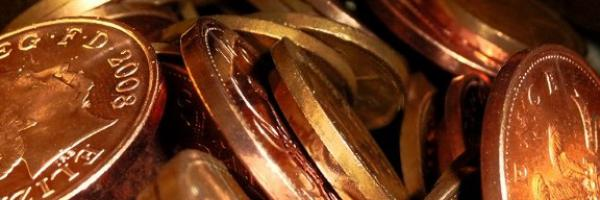# Pennies and TuppencesCharlie won some 1p and 2p coins on the Penny Falls games. When he played around with the coins, he found he could make 8p in five different ways.

Can you find them all? Click below to see:

1p, 1p, 1p, 1p, 1p, 1p, 1p, 1p

1p, 1p, 1p, 1p, 1p, 1p, 2p

1p, 1p, 1p, 1p, 2p, 2p

1p, 1p, 2p, 2p, 2p

2p, 2p, 2p, 2p

Is there a quick way of working out the number of different ways of making any amount with just 1p and 2p coins?

What if we just had 1p and 3p coins instead? Or just 1p and 4p coins? Or ...

Suppose Ways(m,n) describes the number of ways to make a value of n pence using $1$ and $n$ pence coins. Then,
$$\text{Ways}(m,n) = \frac{n + m – n (\mod m)}{m}$$
This works because for any way to make $x$ pence, you can add a $1$ to it and get a way to make $x+1$ pence and the amount of ways to make it doesn’t increase at all. But, if $x$ is a multiple of $m$, a possible way to make $x$ is simply using $m$ pence coins, so the possible ways of making $x$ increases by one.
In my formula, the $m – n (\mod m)$ part creates a number, which when added to $n$, brings it up to the next multiple of m. Finally, the whole result is divided by m to create the amount of ways.